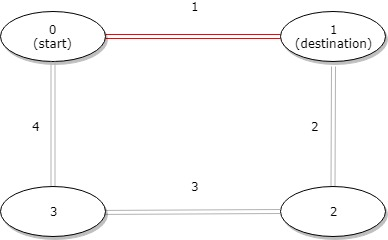### LeetCode - Arrays - Distance between Bus Stops

A bus has `n` stops numbered from `0` to `n - 1` that form a circle. We know the distance between all pairs of neighboring stops where `distance[i]` is the distance between the stops number `i` and `(i + 1) % n`.

The bus goes along both directions i.e. clockwise and counterclockwise.

Return the shortest distance between the given `start` and `destination` stops.

Example 1:```Input: distance = [1,2,3,4], start = 0, destination = 1
Output: 1
Explanation: Distance between 0 and 1 is 1 or 9, minimum is 1.```

```class Solution {     public int distanceBetweenBusStops(int[] distance, int start, int destination) {         int cw_dist = 0;         int acw_dist = 0;         if(start > destination) {             int temp = start;             start = destination;             destination = temp;         }         for(int i=start;i<destination;i++){             cw_dist = cw_dist+distance[i];         }         //System.out.println(cw_dist);                  int j=0;         int counter = destination;         while(j<distance.length){             if(counter > distance.length-1){                 counter = 0;             }             if(counter == start || cw_dist<=acw_dist) break;             acw_dist = acw_dist + distance[counter];             counter++;             j++;         }         //System.out.println(acw_dist);                  return (cw_dist<=acw_dist)?cw_dist:acw_dist;     } }```